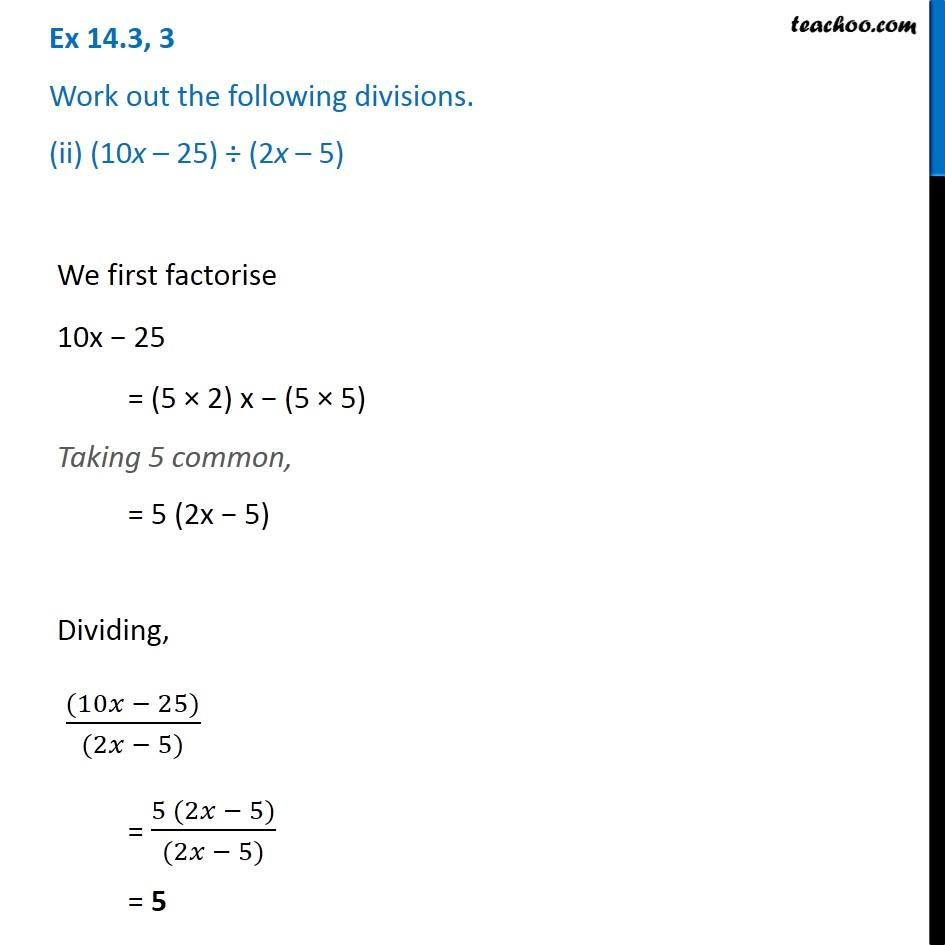1. Chapter 14 Class 8 Factorisation
2. Serial order wise
3. Ex 14.3

Transcript

Ex 14.3, 3 Work out the following divisions. (ii) (10x – 25) ÷ (2x – 5) We first factorise 10x − 25 = (5 × 2) x − (5 × 5) Taking 5 common, = 5 (2x − 5) Dividing, ((10𝑥 − 25))/((2𝑥 − 5)) = (5 (2𝑥 − 5))/((2𝑥 − 5)) = 5

Ex 14.3